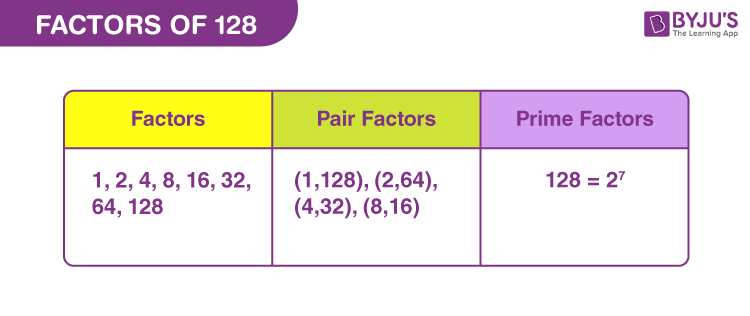# Factors of 128

In Mathematics, factors of 128 are the natural numbers, by which the original number is uniformly divisible. The factors are also called the divisors of 128. 128 is an even composite number, thus it will have more than two factors.

If the product of two numbers results in 128, then such numbers are the factors of 128. 128 is equal to 2 raised to power 7, in exponential form. In this article, we are going to find the factors of 128 along with the pair factors and prime factors, using simple division methods.## How to Find the Factors of 128?

To find the factors of 128, we have to divide 128 by all natural numbers that can evenly divide 128.

• 128 ÷ 1 = 128
• 128 ÷ 2 = 64
• 128 ÷ 4 = 32
• 128 ÷ 8 = 16
• 128 ÷ 16 = 8
• 128 ÷ 32 = 4
• 128 ÷ 64 = 2
• 128 ÷ 128 = 1

Therefore, there are a total sixteen factors of 128: 1, 2, 4, 8, 16, 32, 64 and 128.

## Pair Factors of 128

Pair factors of 128 are the integers, which on multiplication results in the original number.

• 1 × 128 = 128
• 2 × 64 = 128
• 4 × 32 = 128
• 8 × 16 = 128

Therefore, the pair factors of 128 are (1, 128), (2, 64), (4, 32) and (8, 16).

The negative pair factors can be written as the product of two negative numbers that result in 128.

• -1 × -128 = 128
• -2 × -64 = 128
• -4 × -32 = 128
• -8 × -16 = 128

Therefore, the negative pair factors are (-1, -128), (-2, -64), (-4, -32) and (-8, -16).

## Prime Factorisation of 128

The prime factors of 128 can be determined by the prime factorisation method, dividing the original number by prime numbers till the result is 1.

 128 ÷ 2 = 64 64 ÷ 2 = 32 32 ÷ 2 = 16 16 ÷ 2 = 8 8 ÷ 2 = 4 4 ÷ 2 = 2 2 ÷ 2 = 1

Therefore, the prime factor of 128 is 2.

 Prime factorisation of 128 = 2 x 2 x 2 x 2 x 2 x 2 x 2 Exponential form = 27

## Solved Examples

Q.1: What should be multiplied by 32 to get 128?

Solution: Suppose, n is the number to be multiplied by 32 to get 128.

So, n × 32 = 128

n = 128/32

n = 4

Therefore, 4 should be multiplied by 32 to get 128 as the result.

Q.2: What is the sum of factors of 128?

Solution: The factors of number 128 are 1, 2, 4, 8, 16, 32, 64, and 128.

Sum of all the factors = 1 + 2 + 4 + 8 + 16 + 32 + 64 + 128

Sum = 255

Therefore, 255 is the required sum.

Q.2: What is the greatest common factor of 148 and 128?

Solution:

The factors of 128 are 1, 2, 4, 8, 16, 32, 64, 128

Factors of 148 are 1, 2, 4, 37, 74, 148

Therefore, the greatest common factor of 148 and 128 is:

GCF (148, 128) = 4

### Practice Questions

1. What are the divisors of 128?
2. Find the common factors of 128 and 132.
3. What is the GCF of 98 and 128?
4. What is the GCF of 128 and 129?
5. What are the prime factors of 128?

## Frequently Asked Questions on Factors of 128

### What are the factors of 128?

The factors of 128 are 1, 2, 4, 8, 16, 32, 64 and 128.

### What are the multiples of 128?

The first ten multiples of 128 are 128, 256, 384, 512, 640, 768, 896, 1024, 1152 and 1280.

### What is the prime factor form of 128?

The prime factor form of 128 is 2 x 2 x 2 x 2 x 2 x 2 x 2.

### Is 128 a perfect cube?

128 is not a perfect cube. No natural number can be multiplied thrice by itself, to get 128.

### Is 128 a composite number or prime number?

128 is a composite number because it has more than two factors.